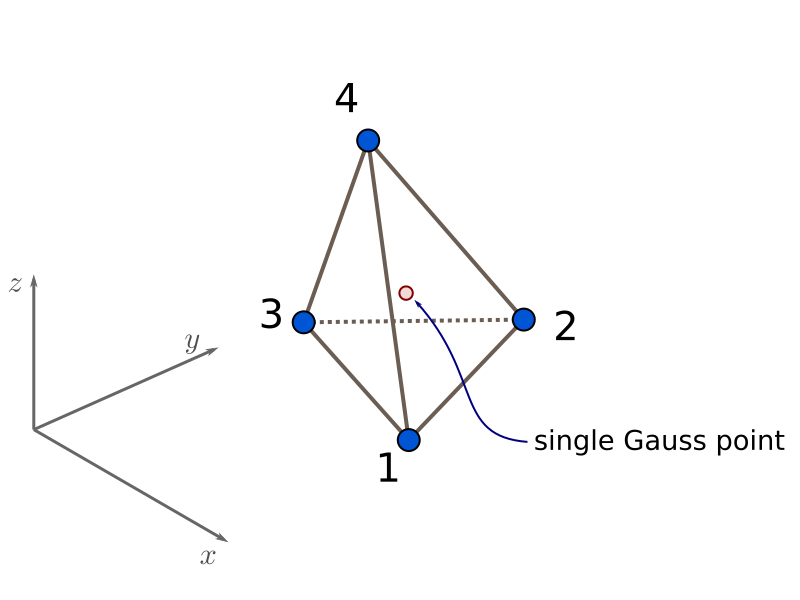# 3.1.9.17. FourNodeTetrahedron Element¶

This command is used to construct an four-node tetrahedron element object, which uses the standard isoparametric formulation.

Command

element FourNodeTetrahedron $eleTag$node1 $node2$node3 $node4$matTag <$b1$b2 $b3> Argument Type Description$eleTag

integer

unique element object tag

$node1 ..$node4

4 integer

nodes of tet (ordered as shown in fig below)

$matTag integer tag of nDMaterial$b1 $b2$b3

list float

optional: body forces in global x y z directions

This is the simplest possible continuum finite element for 3-D analysis. It’s based on linear interpolation of nodal quantities, this means that the strain and stress field inside the element are constant. The single Gauss point results can be interpreted as constant within the element. Because of this, the element has a tendency to lock up when used for simulating bending. In the incompressible limit (caution for materials with $$\nu \rightarrow 0.5$$ or metal plasticity) this element will also lock up. Therefore, caution is warranted when using this element as un-careful mesh refinement might not guarantee a convergence to the mathematical solution or a fast-enough convergence rate. If possible, use a higher-order element like the TenNodeTetrahedron Element.Fig. 3.1.9.17 FourNodeTetrahedron Element Node Numbering

Note

The valid queries to a FourNodeTetrahedron element when creating an ElementRecorder object are ‘forces’, ‘stresses,’ (‘strains’ version > 2.2.0) and ‘material $matNum matArg1 matArg2 …’ Where$matNum refers to the material object at the integration point corresponding to the node numbers in the isoparametric domain.

This element can only be defined after a model Command with -ndm 3 -ndf 3

Example

The following example constructs a FourNodeTetrahedron element with tag 1 between nodes 1, 2, 3, 4 with an nDMaterial of tag 1 and body forces given by varaiables b1, b2, b3.

1. Tcl Code

element FourNodeTetrahedron 1 1 2 3 4 1 $b1$b2 \$b3

1. Python Code

element('FourNodeTetrahedron',1,1,2,3,4,1, b1, b2, b3)


Code Developed by: José Antonio Abell (UANDES). For issues, start a new issue on the OpenSees github repo and tag me (@jaabell).